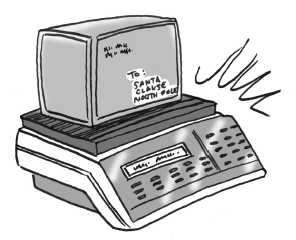### Home > GB8I > Chapter 5 Unit 6 > Lesson INT1: 5.1.2 > Problem5-25

5-25.AAA Packages Plus sends packages overnight for $\5$ plus $\0.25$ per ounce. United Packages charges $\2$ plus $\0.35$ per ounce.

1. Mr. Molinari noticed that his package would cost the same to mail using either service. Write an equation to represent this situation.

2. How much does Mr. Molinari’s package weigh?

Write equations for both brands.

$y=\text{total cost}$
$x=\text{weight in ounces}$

Set the equations equal to each other to solve for $x$.